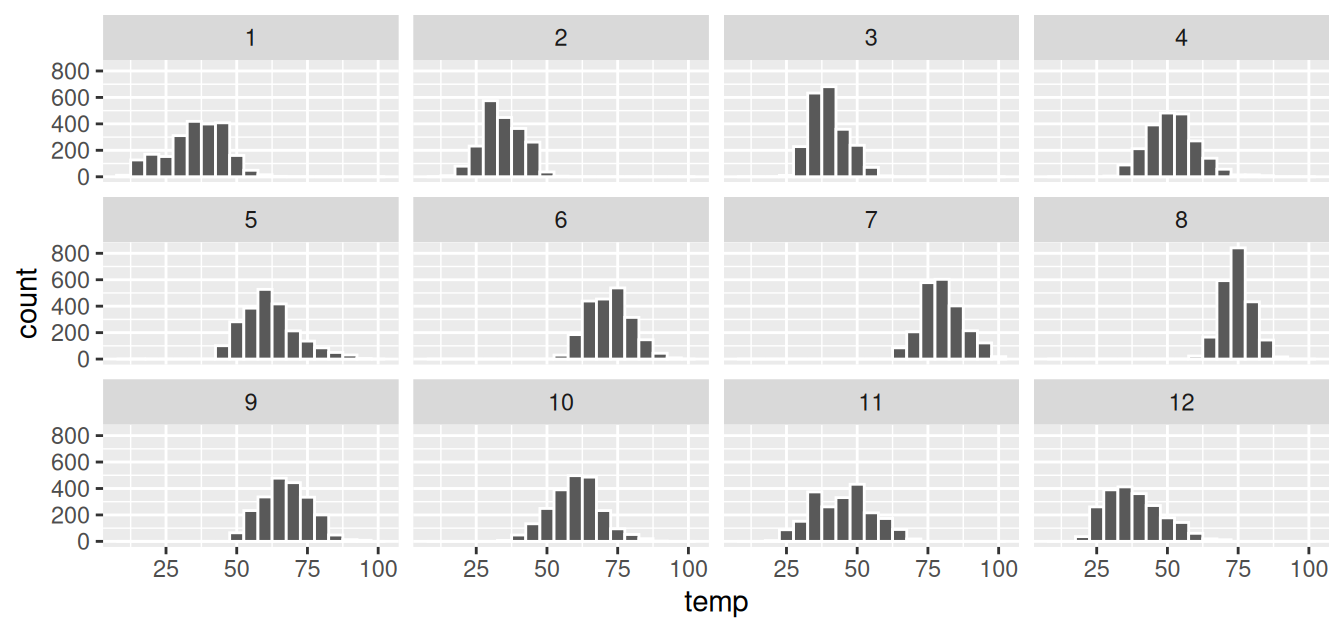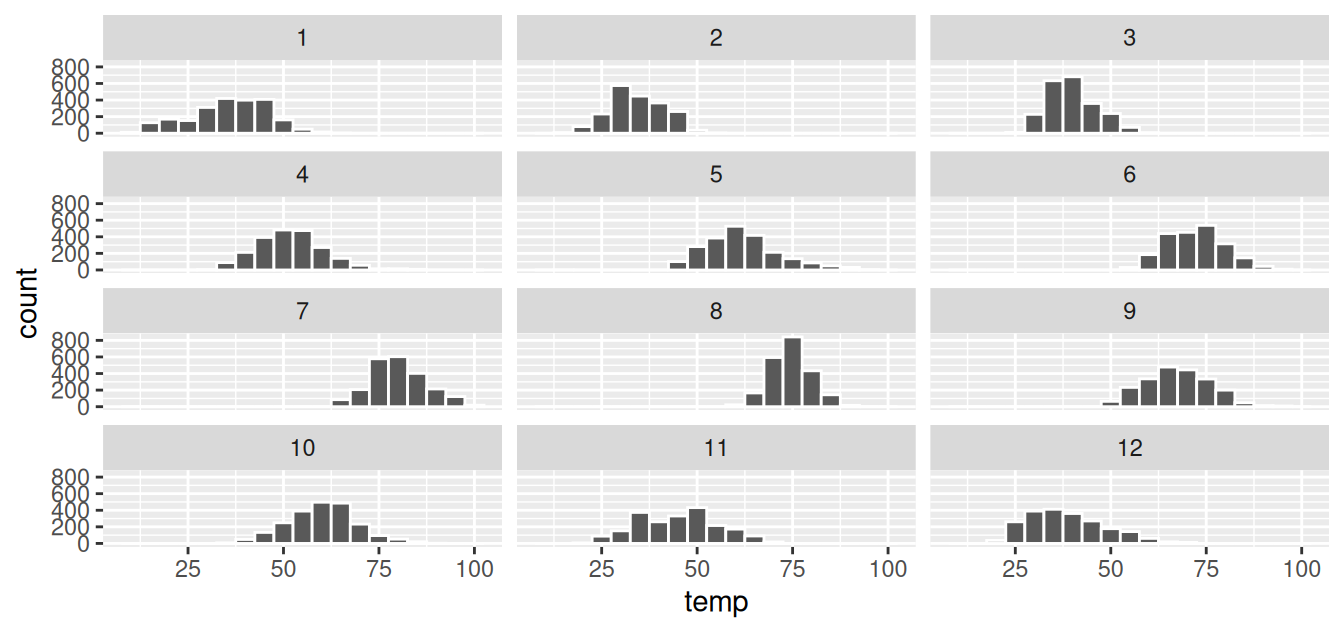## 2.6 Facets

Before continuing with the next of the 5NG, let’s briefly introduce a new concept called faceting. Faceting is used when we’d like to split a particular visualization by the values of another variable. This will create multiple copies of the same type of plot with matching x and y axes, but whose content will differ.

For example, suppose we were interested in looking at how the histogram of hourly temperature recordings at the three NYC airports we saw in Figure 2.9 differed in each month. We could “split” this histogram by the 12 possible months in a given year. In other words, we would plot histograms of temp for each month separately. We do this by adding facet_wrap(~ month) layer. Note the ~ is a “tilde” and can generally be found on the key next to the “1” key on US keyboards. The tilde is required and you’ll receive the error Error in as.quoted(facets) : object 'month' not found if you don’t include it here.

ggplot(data = weather, mapping = aes(x = temp)) +
geom_histogram(binwidth = 5, color = "white") +
facet_wrap(~ month)FIGURE 2.13: Faceted histogram of hourly temperatures by month.

We can also specify the number of rows and columns in the grid by using the nrow and ncol arguments inside of facet_wrap(). For example, say we would like our faceted histogram to have 4 rows instead of 3. We simply add an nrow = 4 argument to facet_wrap(~ month).

ggplot(data = weather, mapping = aes(x = temp)) +
geom_histogram(binwidth = 5, color = "white") +
facet_wrap(~ month, nrow = 4)FIGURE 2.14: Faceted histogram with 4 instead of 3 rows.

Observe in both Figures 2.13 and 2.14 that as we might expect in the Northern Hemisphere, temperatures tend to be higher in the summer months, while they tend to be lower in the winter.

Learning check

(LC2.18) What other things do you notice about this faceted plot? How does a faceted plot help us see relationships between two variables?

(LC2.19) What do the numbers 1-12 correspond to in the plot? What about 25, 50, 75, 100?

(LC2.20) For which types of datasets would faceted plots not work well in comparing relationships between variables? Give an example describing the nature of these variables and other important characteristics.

(LC2.21) Does the temp variable in the weather dataset have a lot of variability? Why do you say that?